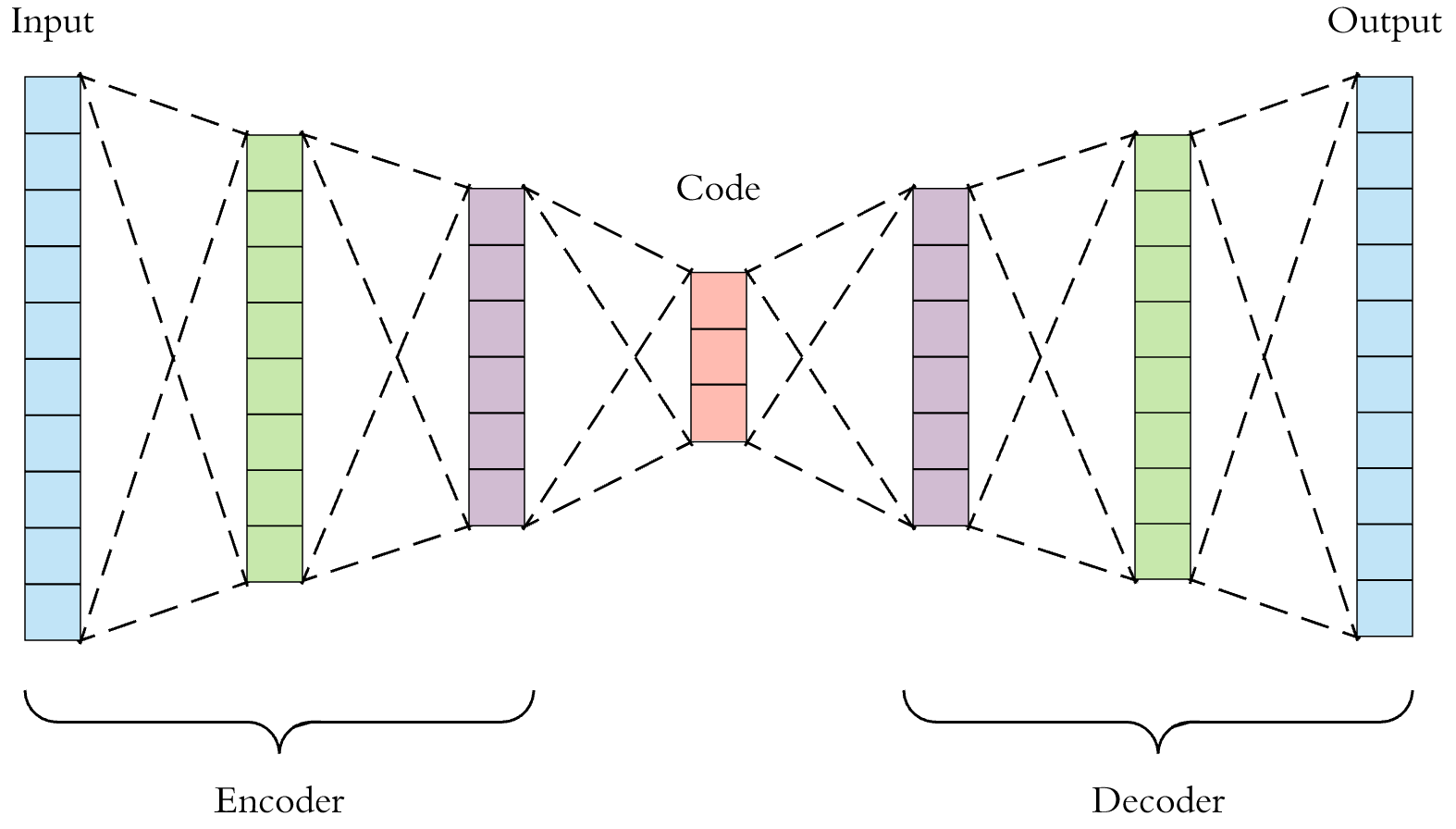/  Deep Learning Interview questions and answers   /  Explain about Under complete Autoencoder?## Explain about Under complete Autoencoder?

Ans: Under complete Autoencoder is a type of Autoencoder. Its goal is to capture the important features present in the data. It has a small hidden layer hen compared to Input Layer. This Autoencoder do not need any regularization as they maximize the probability of data rather copying the input to output. One way to get useful features from the autoencoder is to constrain H to have a smaller dimension than x. An autoencoder whose code dimension is less than the input dimension is called undercomplete. Learning an undercomplete representation forces the autoencoder to capture the most salient features of the training data.

The learning process is described simply as minimizing a loss function

L(x, g(f(x)))

where

L is a loss function penalizing g(f(x)) for being dissimilar from x, such as the mean squared error.

When the decoder is linear and L is the mean squared error, an undercomplete autoencoder learns to span the same subspace as PCA. In this case, an autoencoder trained to perform the copying task has learned the principal subspace of the training data as a side eﬀect.

Autoencoders with nonlinear encoder functions f and nonlinear decoder functions

G can thus learn a more powerful nonlinear generalization of PCA. Unfortunately, if the encoder and decoder are permitted too much capacity, the autoencoder can learn to perform the copying task without extracting useful information about the distribution of the data.